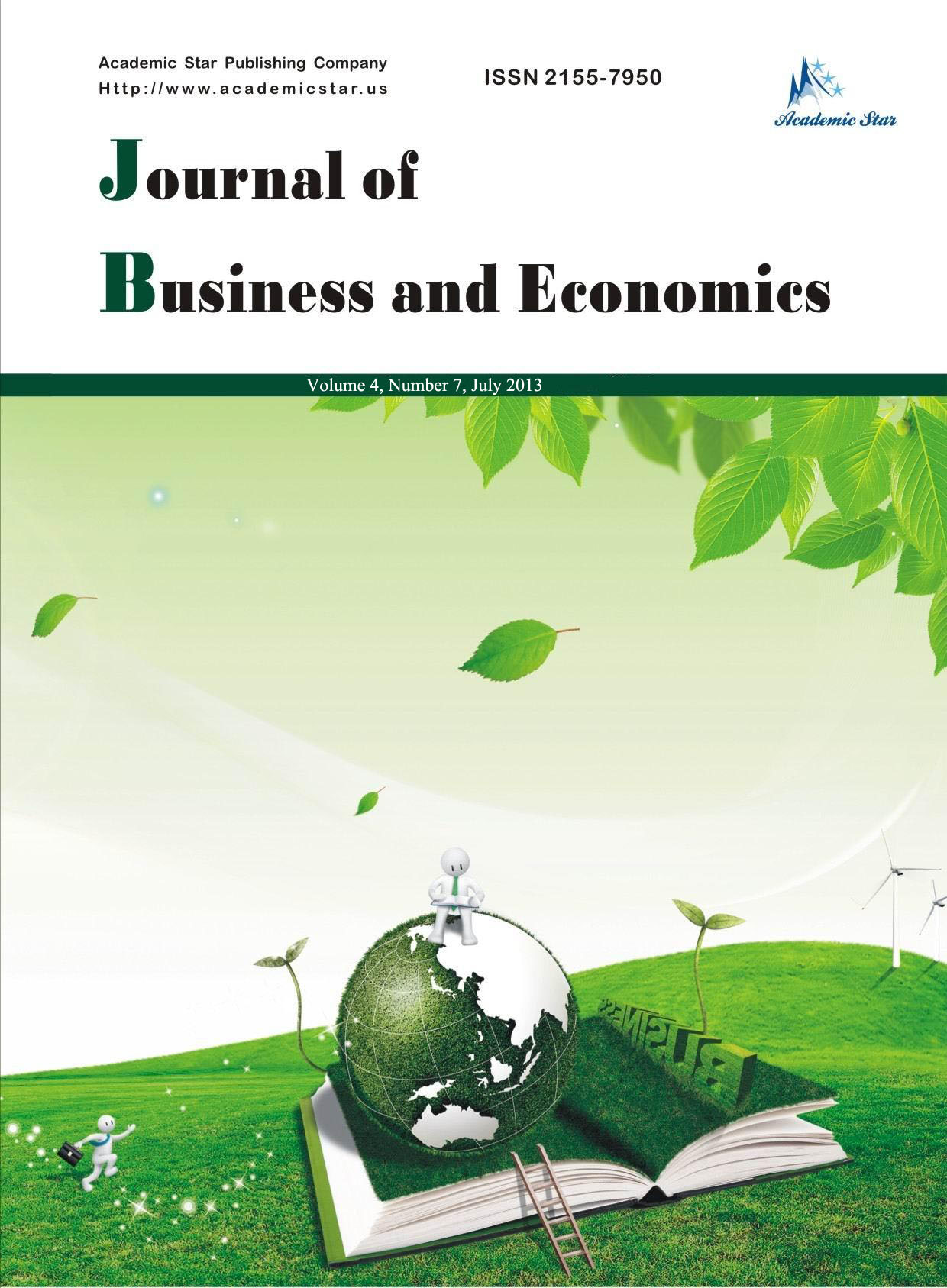Economics• ISSN: 2155-7950
• Journal of Business and Economics

Estimation of Money Demand Function in Somalia: Cointegration and Vector Error Correction Model

Abdinur Ali Mohamed, Ahmed Ibrahim Nageye
(Faculty of Economics, SIMAD University, Somalia)

Abstract: We used vector error correction model to estimate money demand function in Somalia using time series data covering 1970-2010. Data was collected from world development indicators and the Fred Economic Data.

Ordinary least square was used to measure the coefficients of the money demand determinants. Real money supply was the dependent variable where GDP, inflation rate, exchange rate, purchasing power and the foreign interest rate, were the explanatory variables of this study. Augmented Dickey-Fuller test was used to examine the unit root of the data. Intercept and the trend were used while testing the unit root. Johansen Cointegration was employed to determine if the variables are cointegrated in the long run and move together. Vector Error Correction was used to study the causality between the study variables.

The study found that five out of the six variables were different from zero, meaning that they are statistically significant. Purchasing power tends to be negative, indicating that the decrease of the money value leads the money demand to increase.

The unit root of the data in the all variables was tested and the variables happen to be none stationary at the level, but, stationary at the first difference I(1). The null hypothesis of no cointegration was rejected using Johansen Cointegration.

Key words: money demand; scale variable; permanent income hypothesis; granger causality

JEL codes: E41